Importance: High ✭✭✭
 Author(s): Woodall, Douglas R.
 Subject: Graph Theory » Directed Graphs
 Keywords: digraph packing
 Prize: \$5000 (Cornuejols [C])
 Posted by: mdevos on: April 5th, 2007
Conjecture   Ifis a directed graph with smallest directed cut of size, thenhasdisjoint dijoins.

Definitions: Letbe a directed graph. A directed cut ofis an edge-cut in which all edges are directed the same way. A dijoin is a set of edges which intersect every directed cut.

There is an important theorem of Lucchesi and Younger [LY] which asserts that the dual problem has an optimum integer packing. That is, for every digraphin which the smallest dijoin has size, there existpairwise disjoint directed cuts. This result implies more generally (in the terminology of Corneujols [C]) that the clutter of (minimal) directed cuts has the max-flow min-cut property (MFMC). It follows from this and Lehman's theorem [L] that the clutter of (minimal) dijoins is ideal. Therefore, if the smallest directed cut ofhas size, then there exist nonnegative rational numberssumming toand dijoins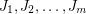so that if each dijoin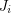is taken with weight, the total weight of the dijoins containing any edge is at most 1. The above conjecture asserts that such a combination exists withintegral.

Schrijver [Sc80] has found a digraph in which the clutter of (minimal) dijoins does not have the MFMC property. Thus, the weighted version of Woodall's conjecture is not true in general. However, this clutter does have the MFMC property in a number of interesting special cases. One such example (due to Schrijver [Sc82] and Feofiloff, Younger [FY]) is when the digraph is acyclic and every source is joined to every sink by a directed path. Since every such digraph has the MFMC property, every such digraph must satisfy Woodall's conjecture.

There seem to be few positive results towards Woodall's conjecture for general digraphs. Although this was probably already known, Seymour and I (M. DeVos) observed that the conjecture is true for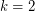. To see this, note that the underlying graph is 2-edge-connected, so it may be oriented to give a strongly connected digraph, call it. Now partition the edges into two sets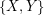whereconsists of those edges which have the same orientation in bothand, andconsists of those edges with different orientations in the two graphs. It is immediate that bothandmeet every directed cut, so each is a dijoin. Extending this toappears difficult. Indeed, I believe the following weak version of this is still open.

Conjecture   Prove that there exists a fixed integerso that every digraph with all directed cuts of sizecontains three pairwise disjoint dijoins.

The restriction of this conjecture to the special case of planar graphs is also open. Here we can use duality to restate the conjecture. Call a set of edgesa feedback arc-set ifintersects every directed cycle (or equivalently,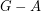is acyclic).

Conjecture   Ifis a planar digraph with all directed cycles of length, thencontainspairwise disjoint feedback arc-sets.

The above conjecture is known to fail when the assumption of planarity is removed. On the other hand, it does hold for digraphs which are orientations of series-parallel graphs [LW]. It is also still open for.

## Bibliography

[C] G. Cornuejols, Combinatorial Optimization, Packing and Covering SIAM, Philadelphia (2001). MathSciNet

[FY] P. Feofiloff and D. H. Younger, Directed cut transversal packing for source-sink connected graphs. Combinatorica 7 (1987), no. 3, 255--263. MathSciNet

[LW] O. Lee, Y. Wakabayashi, Note on a min-max conjecture of Woodall. J. Graph Theory 38 (2001), no. 1, 36--41. MathSciNet

[LY] C.L. Lucchesi and D. H. Younger A minimax theorem for directed graphs, Journal of the London Math. Soc. (2) 17 (1978) 369-374. MathSciNet

[Sc80] A. Schrijver, A counterexample to a conjecture of Edmonds and Giles, Discrete Math. 32 (1980) 213-214. MathSciNet

[Sc82] A. Schrijver, Min-max relations for directed graphs, Annals of Discrete Math. 16 (1982) 261-280. MathSciNet

[W] D. R. Woodall, Menger and König systems. Theory and applications of graphs (Proc. Internat. Conf., Western Mich. Univ., Kalamazoo, Mich., 1976), pp. 620--635, Lecture Notes in Math., 642, Springer, Berlin, 1978. MathSciNet

* indicates original appearance(s) of problem.